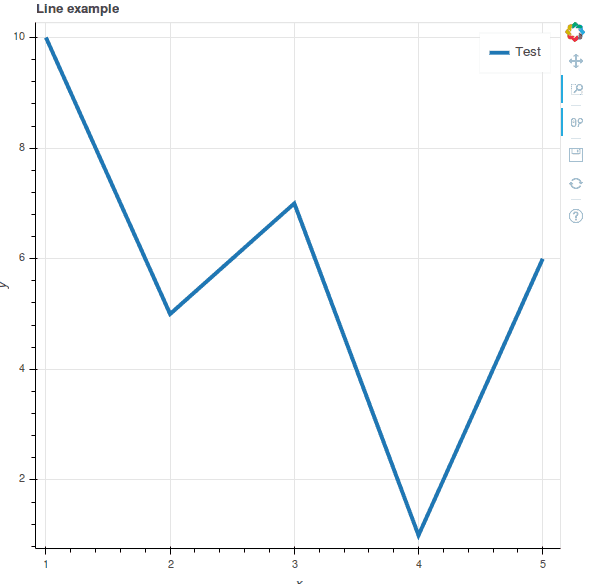# Python: Visualization with Bokeh

The Bokeh package is an interactive visualization library that uses web browsers for its presentation. Its goal is to provide graphics in the vein of D3.js that look elegant and are easy to construct. Bokeh supports large and streaming datasets. You will probably be using this library for creating plots / graphs. One of its primary competitors seems to be Plotly.

Note: This will not be an in-depth tutorial on the Bokeh library as the number of different graphs and visualizations it is capable of is quite large. Instead, the aim of the article is to give you a taste of what this interesting library can do.

Let’s take a moment and get it installed. The easiest way to do so is to use pip or conda. Here’s how you can use pip:

```pip install bokeh
```

This will install Bokeh and all its dependencies. You may want to install Bokeh into a virtualenv because of this, but that’s up to you. Now let’s check out a simple example. Save the following code into a file with whatever name you deem appropriate.

```from bokeh.plotting import figure, output_file, show

output_file("/path/to/test.html")

x = range(1, 6)
y = [10, 5, 7, 1, 6]
plot = figure(title='Line example', x_axis_label='x', y_axis_label='y')
plot.line(x, y, legend='Test', line_width=4)
show(plot)
```

Here we just import a few items from the Bokeh library. We just tell it where to save the output. You will note that the output is HTML. Then we create some values for the x and y axises so we can create the plot. Then we actually create the figure object and give it a title and labels for the two axises. Finally we plot the line, give it a legend and line width and show the plot. The show command will actually open your plot in your default browser. You should end up seeing something like this:Bokeh also supports the Jupyter Notebook with the only change being that you will need to use output_notebook instead of output_file.

The Bokeh quick start guide has a neat example of a series of sine waves on a grid plot. I reduced the example down a bit to just one sine wave. Note that you will need NumPy installed for the following example to work correctly:

```import numpy as np

from bokeh.layouts import gridplot
from bokeh.plotting import figure, output_file, show

N = 100
x = np.linspace(0, 4*np.pi, N)
y0 = np.sin(x)

output_file('sinewave.html')

sine = figure(width=500, plot_height=500, title='Sine')
sine.circle(x, y0, size=10, color="navy", alpha=0.5)

p = gridplot([[sine]], toolbar_location=None)

show(p)
```

The main difference between this example and the previous one is that we are using NumPy to generate the data points and we’re putting our figure inside of a gridplot instead of just drawing the figure itself. When you run this code, you should end up with a plot that looks like this:If you don’t like circles, then you’ll be happy to know that Bokeh supports other shapes, such as square, triangle and several others.

### Wrapping Up

The Bokeh project is really interesting and provides a simple, easy-to-use API for creating graphs, plots and other visualizations of your data. The documentation is quite well put together and includes lots of examples that showcase what this package can do for you. It is well worth just skimming the documentation so you can see what some of the other graphs look like and how short the code examples are that generate such nice results. My only gripe is that Bokeh doesn’t have a way to save an image file programmatically. This appears to be a long term bug that they have been trying to fix for a couple of years now. Hopefully they find a way to support that feature soon. Otherwise, I thought it was really cool!

### 2 thoughts on “Python: Visualization with Bokeh”

1. Pingback: Linkdump #6 | WZB Data Science Blog